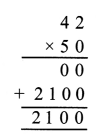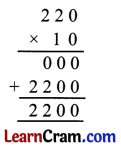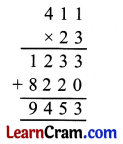# DAV Class 4 Maths Chapter 3 Worksheet 1 Solutions

The DAV Class 4 Maths Book Solutions and DAV Class 4 Maths Chapter 3 Worksheet 1 Solutions of Multiplication offer comprehensive answers to textbook questions.

## DAV Class 4 Maths Ch 3 WS 1 Solutions

Question 1.
Multiply
(a) 317 × 125(b) 892 × 243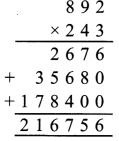(c) 734 × 162(d) 931 × 217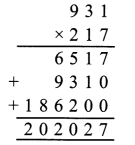(e) 753 × 135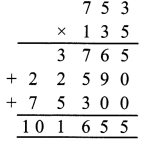(f) 731 × 307Question 2.
Multiply.
(a) 431 by 721(b) 821 by 621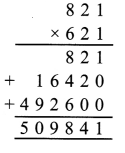(c) 972 by 340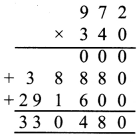(d) 435 by 425(e) 1,432 by 211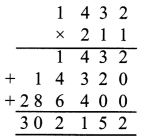(f) 7,312 by 135Question 3.
Find the product.
(a) 437 × 211(b) 713 × 217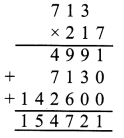(c) 982 × 133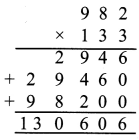(d) 345 × 264(e) 1,732 × 259(f) 1,083 × 847Question 4.
Using the digits 3, 1 and 5 only once, write the smallest and the largest 3-digit numbers. Also find their product.
Smallest 3-digit number = 135
Largest 3-digit number = 531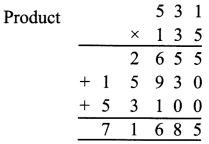Thus, the product of largest and smallest 3-digit numbers is 71,685.

### DAV Class 4 Maths Chapter 3 Worksheet 1 Notes

The number which has to be multiplied is called multiplicand. The number by which we multiply is called multiplier and the answer we get after multiplication is called product.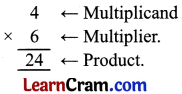• If we multiply two or three numbers in any order, the product remains the same.
• The product of a number with 1 is the number itself.
• The product of any number with 0 is zero.

Multiplication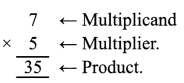Multiplication (3-digit and 4-digit)
E.g. Multiply 2,135 by 127Review Exercise

Solve some problems

Question 1.
Multiply.
(a) 76 × 13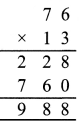(b) 532 × 25(c) 127 × 43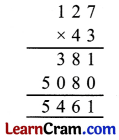(d) 483 × 21Question 2.
Multiply.
(a) 32 by 2(b) 101 by 5(c) 98 by 121(d) 213 by 42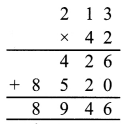Question 3.
Find the product.
(a) 713 × 3